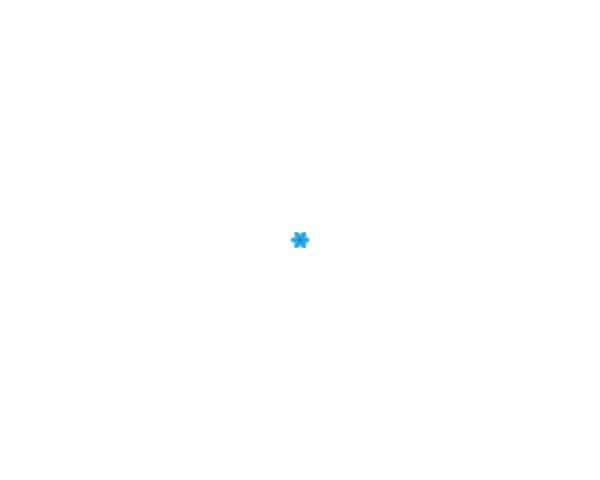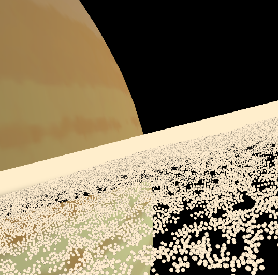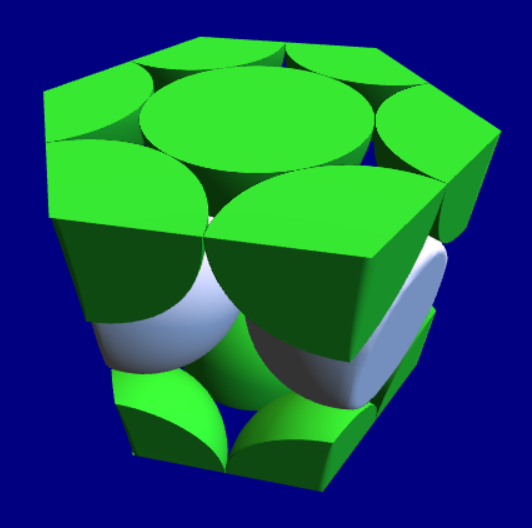﻿ Maths Exam

### Maths Exam

1. Solution of linear equations

 We have the following equations: $$\begin{eqnarray} -2 x +y &=& 3 \\ 3 x -6 y &=& 2 \end{eqnarray}$$ Correct Answer $$x=\frac{-20}{9}, y=\frac{-13}{9}$$

2. Differentiation A

 Differentiate the following equation: $$\sqrt{\ln\left({x}^{2}\right)}$$Correct Answer $$\frac{1}{x\sqrt{\ln\left({x}^{2}\right)}}$$

3. Differentiation A

 Differentiate the following equation: $$\ln\left({x}^{2} + \sin\left(x\right)\right)$$Correct Answer $$\frac{2x + \cos\left(x\right)}{{x}^{2} + \sin\left(x\right)}$$

4. Differentiation B

 Differentiate the following equation: $$\frac{2x\sin\left(x\right)}{\ln\left(\cos\left(x\right)\right)}$$Correct Answer $$\frac{2\left(\sin\left(x\right) + x\cos\left(x\right)\right)}{\ln\left(\cos\left(x\right)\right)} - \frac{-2x{\sin\left(x\right)}^{2}}{{\ln\left(\cos\left(x\right)\right)}^{2}\cos\left(x\right)}$$

5. Integration

 Please carry out the following integral: $$\int \sqrt{x - 4} dx$$Correct Answer $$\frac{2{\left(x - 4\right)}^{\frac{3}{2}}}{3}$$

6. Integration

 Please carry out the following integral: $$\int {\left(2x\right)}^{1} dx$$Correct Answer $${x}^{2}$$

7. Consider the following differential equation: $$3\frac{d^2y}{dx^2} + 3\frac{dy}{dx} - 6y = 0$$

 Find the general solution to the above equation.Correct Answer $$A\exp\left(x\right) + B\exp\left(-2x\right)$$

8. Consider the following series: $$\sum_{j=0}^{11} (-\left(j - 6\right))$$

 What does the sum evaluate to?Correct Answer $$6$$

9. Solution of linear equations

 We have the following equations: $$\begin{eqnarray} 6 x -5 y &=& 1 \\ 3 x -3 y &=& 4 \end{eqnarray}$$ Correct Answer $$x=\frac{-17}{3}, y=-7$$

10. Differentiation A

 Differentiate the following equation: $$\sqrt{\cos\left(x\right)} + \sqrt{5x}$$Correct Answer $$\frac{(-\sin\left(x\right))}{2\sqrt{\cos\left(x\right)}} + \frac{\sqrt{5}}{2\sqrt{x}}$$

## Other Articles:## How to simulate fractal ice crystal growth in Python

This presents python code to draw snowflakes, simulating a diffusion process with Fourier transforms.## Saturn's rings

A simulation of Saturn's rings --- a few thousand particles are simulated, in a repeating tiled region. You use the mouse and keys to fly in it.

## Three Crystal Structures

A ThreeJS model of three crystal structures, by Dr. Jennifer Martay.